Courses

# NCERT Exemplar - Atoms and Molecules (part-2) Class 9 Notes | EduRev

## Class 9 : NCERT Exemplar - Atoms and Molecules (part-2) Class 9 Notes | EduRev

The document NCERT Exemplar - Atoms and Molecules (part-2) Class 9 Notes | EduRev is a part of the Class 9 Course Science Class 9.
All you need of Class 9 at this link: Class 9

Q.16. Calcium chloride, when dissolved in water, dissociates into its ions according to the following equation.
CaCl2 (aq) → Ca2+ (aq) + 2CI- (aq)
Calculate the number of ions obtained from CaCl2 when 222 g of it is dissolved in water.
Ans.
CaCl2(aq) → Ca2+(aq) + 2Cl(aq)
1 mole of CaCl2 = 111 g
111 g of CaCl2 = 1 mole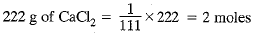1 mole of CaCl2 gives 3 moles of ion
2 moles of CaCl2 gives 6 moles of ion
= 6 x 6.022 x 1023 ions
= 36.132 x 1023 ions = 3.6132 x 1024 ions

Q.17. The difference in the mass of 100 moles each of sodium atoms and sodium ions is 5.48002 g.
Compute the mass of an electron.
Ans.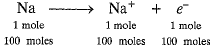100 moles of electrons weigh = 5.48002 g
100 x 6.022 x 1023 electrons weigh = 5.48002 g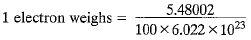= 0.91 x 10-25g = 9.1 x 10-26g = 9.1 x 10-29kg
It is not the real mass of electron.

Q.18. Cinnabar (HgS) is a prominent ore of mercury. How many grams of mercury are present in 225 g of pure HgS? Molar mass of Hg and S are 200.6 g mol-1 and 32 g mol-1 respectively.
Ans.
Molar mass of HgS = 200.6 + 32
= 232.6 g mol-1
232.6 g of HgS contains 200.6 g of pure Hg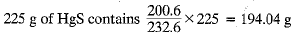Q.19. The mass of one steel screw is 4.11 g. Find the mass of one mole of these steel screws. Compare this value with the mass of the Earth (5.98 x 1024 kg). Which one of the two is heavier and by how many times?
Ans.
Mass of 1 steel screw = 4.11 g
Mass of 6.022 x 1023 steel screws
= 4.11 x 6.022 x 1023 g = 2.475 x 1024 g
= 2.475 x 1021 kg
Therefore, mass of one mole of screws
= 2.475 x 1021 kg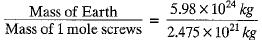= 2.4 x 103
Mass of the earth is 2400 times the mass of 1 mole of screws.

Q.20. A sample of vitamin C is known to contain 2.58 x 1024 oxygen atoms. How many moles of oxygen atoms are present in the sample?
Ans.
Number of moles of oxygen atoms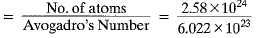= 4.28 moles.

Q.21. Raunak took 5 moles of carbon atoms in a container and Krish also took 5 moles of sodium atoms in another container of the same weight.
(a) Whose container is heavier?
(b) Whose container has more number of atoms?
Ans.
(a) 1 mole of carbon atoms = 12 g
5 moles of carbon atoms = 12 x 5 = 60 g
1 mole of Na = 23 g
5 moles of Na = 23 x 5 = 115 g
Thus, Krish’s container is heavier than that of Raunak.
(b) Both the containers have same number of atoms as both have the same number of moles and both elements are monoatomic.

Q.22. Fill in the missing data in the Table.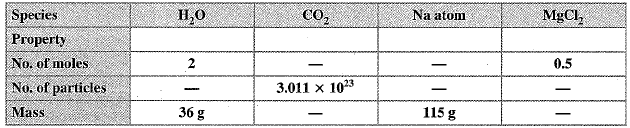Ans.
1 mole of H2O = 1 x 2 + 16 = 18 g
2 moles of H20 = 2 x 18 = 36 g
1 mole of H20 contains 6.022 x 1023 molecule
2 moles of H20 contains 2 x 6.022 x 1023 = 12.044 x 1023 molecules
1 mole of CO2 = 12 + 2 + 16 = 44 g
0.5 mole of CO2 = 44 x 0.5 = 22 g
1 mole of CO2 contains 6.022 x 1023 molecules
0.5 mole of CO2 will contain 0.5 x 6.022 x 1023 = 3.011 x 1023 molecules
1 mole of Na = 23 g
5 moles of Na = 23 x 5 = 115 g
1 mole of Na = 6.022 x 1023 atoms
5 moles of Na = 5 x 6.022 x 1023 = 30.110 x 1023 atoms
1 mole of MgCl2 = 24 + 2 x 35.5 = 24 + 71 = 95 g
0.5 mole of MgCl= 0.5 x 95 = 47.5 g
1 mole of MgCl2 = 6.022 X 1023
0.5 mole of MgCl2 = 0.5 x 6.022 x 1023 = 3.011 x 1023 formula units.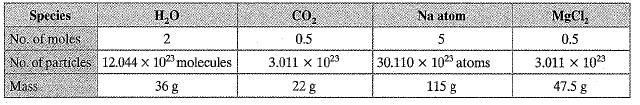Q.23. The visible universe is estimated to contain 1022 stars. How many moles of stars are present in the visible universe?
Ans. Number of moles of stars =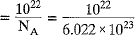= 0.167 x 10-1 = 0.0167 mole.

Q.24. What is the SI prefix for each of the following multiples and sub-multiples of a unit?
(a) 103
(b) 10-1
(c) 10-
(d) 10-6
(e) 10-9
(f) 10-12
Ans.
(a) kilo
(b) deci
(c) centi
(d) micro
(e) nano
(f) pico

25. Express each of the following in kilograms:
(a) 5.84 x 10-3 mg
(b) 58.34 g
(c) 0.584 g
(d) 5.873 x 10-21g
Ans.
(a) 5.84 x 10-3 mg x 10-6 = 5.84 x 10-9 kg [∵ 1 mg = 10-3 g = 10-6 kg]
(b) 58.34 g x 10-3 kg = 5.834 x 10-2 kg [∵ 1 g = 10-3 kg]
(c) 0.584 g = 0.584 x 10-3 kg = 5.84 x 10-4 kg
(d) 5.873 x 10-21 g x 10-3 = 5.873 x 10-24 kg

Q.26. Compute the difference in masses of 103 moles each of magnesium atoms and magnesium ions. (Mass of an electron = 9.1 x 10-31 kg)
Ans.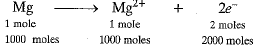1 electron weighs = 9.1 x 10-31 kg
∴ 2000 x 6.022 x 1023 electrons weigh
= 9.1 x 10-31 x 2000 x 6.022 x 1023 kg
= 109.6004 x 10-5 kg = 1.096004 x 10-3 kg

Q.27. Which has more number of atoms? 100 g of N2 or 100 g of NH3
Ans.
1 mole of N2 - 28 g
28 g of N2 = 1 mole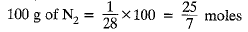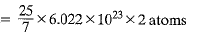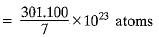= 43.01 x 1023 atoms
= 4.30 x 1024 atoms
1 mole of NH3 = 17 g
17 g of NH3 = 1 mole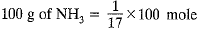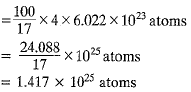Therefore, 100 g of NH3 contains more number of atoms.

Q.28. Compute the number of ions present in 5.85 g of sodium chloride.
Ans.
NaCl → Na+ + Cl-
1 mole of NaCl = 23 + 35.5 = 58.5 g
58.5 g of NaCl = 1 mole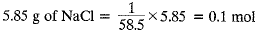1 mole of NaCl gives 2 moles of ions
0.1 mol of NaCl gives 2 x 0.1 = 0.2 mol
= 0.2 x 6.022 x 1023 ions = 1.2044 x 1023 ions

Offer running on EduRev: Apply code STAYHOME200 to get INR 200 off on our premium plan EduRev Infinity!

## Science Class 9

95 videos|223 docs|135 tests

,

,

,

,

,

,

,

,

,

,

,

,

,

,

,

,

,

,

,

,

,

;